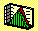2.6 SAMPLE STATISTICS: UNBIASEDNESS

In sample statistics we want sample estimates of population parameters to be unbiased estimators of the population parameters.

An estimate of a population parameter is unbiased if the expected estimation error is zero. Unbiased forecasts are those that have an expected (or ex ante) forecast error of zero. Ex post this error can be non-zero, but if a forecast experiment is conducted for a very large number of trials, the average error from an unbiased forecast is zero.

For the case of a sample drawn randomly from some population of values, the sample mean M is an unbiased forecast of the population mean if and only if the expectation operator applied to M equals the population mean. This is indeed the case for a random sample, because the expected value of each observation in the sample is the population mean, and the average of all these expected values equal N times the population mean divided by N.

The unbiasedness property breaks down, however, in the case of a sample variance. To see why, recall that unbiasedness requires that the expected sample variance equals the true population variance. Consider the definition of a sample variance: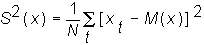By expanding this equation and taking the 1/N average we have: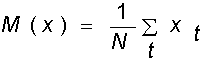and taking expectations yields: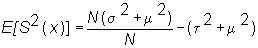where s2 is the population variance of x, and t2 is the variance of the sample mean. Finally, m is the population mean. Thus the expected sample variance is not an unbiased estimate of the population variance because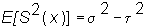There is a simple correction to transform the sample variance into an unbiased estimator of the population variance parameter. This transformation is immediate after observing that: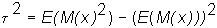or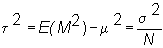and therefore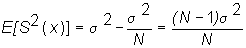Thus the unbiased estimate for the population variance is: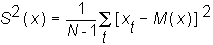In the next topic we introduce the property, relative efficiency.

previous topic

next topic